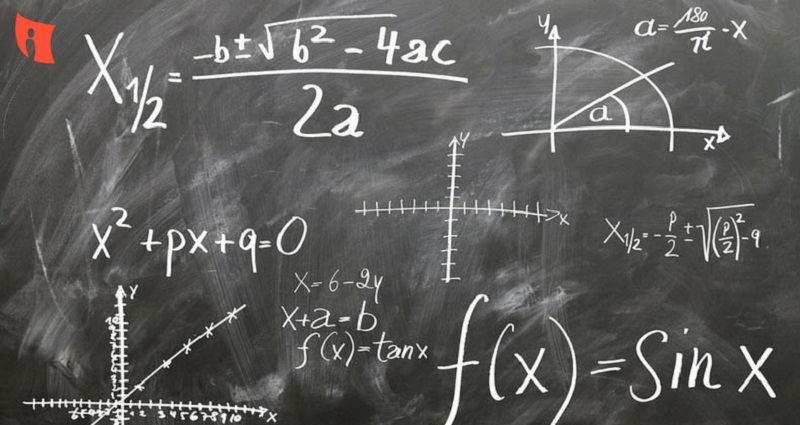# Famous Indian Mathematicians and Their Contributions

Oct 20, 2016Mathematics owes a great deal to the contributions made by Indian mathematicians over many centuries. Indian mathematicians of the early Indus Valley Civilization to the scholars of the 5th to 12th century AD made contributions in the field of algebra, arithmetic, geometry, trigonometry, and differential equations. Later on, in the 14th to 16th AD, Indian mathematicians derived infinite series and expansion of trigonometric functions. In a nutshell, Indian scholars have always led the development of mathematics from the front.

Apart from this, Indian mathematicians are also responsible for the creation, and refinement of the current decimal place-value system, including the number zero, without which higher mathematics would not be possible. In the words of Laplace, the celebrated French scholar who made significant contributions to mathematics –“It is India that gave us the ingenious method of expressing all numbers by means of ten symbols, each symbol receiving a value of position as well as an absolute value; a profound and important idea which appears so simple to us now that we ignore its true merit. But its very simplicity and the great ease which it has lent to computations put our arithmetic in the first rank of useful inventions; and we shall appreciate the grandeur of the achievement the more when we remember that it escaped the genius of Archimedes and Apollonius, two of the greatest men produced by antiquity.

Most famous Indian mathematicians

Here is a look at some of the most famous Indian mathematicians, and their contributions to mathematics.

Baudhayana (800BC)

Baudhayana discovered the Pythagoras Theorem around 1000 years before Pythagoras was even born. In this book, Baudh?yana ?ulbasûtra (800 BC), he wrote, “A rope stretched along the length of the diagonal produces an area which the vertical and horizontal sides make together”. This is nothing, but a different way of looking at Pythagoras theorem. Apart from this, the book contained geometric solutions of a linear equation in a single unknown.

Aryabhata is undoubtedly the most celebrated Indian mathematicians. His most significant contributions to mathematics include approximation of the value of pi up to five decimal places, and he also discussed the concept of sine. Aryabhata was the one who calculated the area of the triangle as perpendicular multiplied by the half side. He was the one to calculate that the time that Earth takes to complete one rotation is 365 days. In algebra, he summed series of squares and cubes and solved equations of the type ax -by = c.Brahamagupta is the man who gave the world the concept of negative numbers and zero. He also proposed rules for solving simultaneous and quadratic equations. He calculated the area of a cyclic quadrilateral with semi-perimeter (s). Brahamagupta is the founder of “Numerical Analysis”, a branch of higher mathematics. He was the one to identify that x²- y² = (x+y)(x-y).Bhaskara expanded on the work of Aryabhata, and found an approximation of the sine function. Bhaskara laid the foundation of differential calculus, and gave an example of the differential coefficient and discussed the idea of what we know as Rolle’s Theorem today. He told the world that sum of any number and infinity is infinity, and any number divided by zero is infinity. He was the one to introduce the cyclic method of solving algebraic equations. The inverse cyclic” method that we know today stems from this.

(800-870 A.D)

Mahavira was a Jain mathematician, who derived the volume of frustum by an infinite procedure. He worked with logarithms in base 2, base 3 and base 4. He authored the book Ganita Sara Samgraha in 850 AD, which included teachings of Brahamagupta, but also contained simplifications and some additional information. It includes chapters on arithmetical operations, mixed operations, operations involving functions, operations relating to calculation of areas and others.

Varahamihira was a mathematician, astrologer, and astronomer. His prominent mathematical work includes the discovery of the following trigonometric formulas:

Varahamihira also improved the accuracy of Aryabhatta’s sine tables. Varahamihira also defined the algebraic properties of zero and negative numbers. He was among the first mathematicians to discover a version of Pascal’s triangle as we know it today.

Bhaskara II was a prominent mathematician and astronomer, who proved that any number divided by zero is infinity. He also found that a positive number has two square roots. Bhaskara II was the one to discover the differential coefficient and derivative. He gave the formula

Bhaskara authored six books on mathematics.

Srinivasa Aaiyangar Ramanujan (1887-1920)

Ramanujan is probably the best-known mathematicians of modern India. Some of his most credible contributions to the world of mathematics are the Hardy-Ramanujan-Littlewood circle method, elliptic functions, work on the algebra of inequalities, partial sums and products of hypergeometric series, Roger-Ramanujan’s identities in the partition of numbers and continued fractions. 1729 is known as the Ramanujan number.

Dattaraya Ramchandra Kaprekar (1905–1986)

D.R. Kaprekar was a school teacher in Nashik, and never received a master’s degree. He was often mocked at by his contemporaries, who found his work too trivial. However, today, he is known as one of the most renowned and respected mathematicians of modern India. He is best recognised for the ‘Kaprekar constant’. Take a four digit number with all different digits, arrange the numbers in descending order. Then arrange all numbers in ascending order. Subtract the smaller number from the bigger number, and repeat the process at least seven times. You will get the number 6174 as a result, which is the ‘Kaprekar constant’. He also discovered Self-numbers, Harshad numbers, and more.